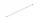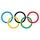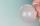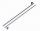Expressions

Find out value of expressions if a = -1, b =2:

x=b - 2a - ab
y=a3 - b2 - 2ab
z=a2 b3 - a3 b2
w=a + b + a3 - b2

Result

x =  6
y =  -1
z =  12
w =  -4

Solution:Leave us a comment of this math problem and its solution (i.e. if it is still somewhat unclear...):Be the first to comment!Next similar math problems:

1. Valid numberRound the 453874528 on 2 significant numbers.
2. Flood waterFlood waters in some US village meant that the homes had to evacuate 364 people. 50 of them stayed at elementary schools, 59 them slept with their friends and others went to relatives. How many people have gone to relatives?
3. Math classificationIn 3A class are 27 students. One-third got a B in math and the rest got A. How many students received a B in math?
4. The resultHow many times I decrease the number 1632 to get the result 24?
5. On the tripOn the trip were more than 55 children, but less than 65 children. Groups of 7 could be formed, but groups of 8 no. How many children were on a trip?
6. Addition of Roman numbersAdded together and write as decimal number: LXVII + MLXIV
7. If-then equationIf 5x - 17 = -x + 7, then x =
8. LineHow many parts of line divide 5 (different) points that lie on it?
9. CagesHonza had three cages (black, silver, gold) and three animals (guinea pig, rat and puppy). There was one animal in each cage. The golden cage stood to the left of the black cage. The silver cage stood on the right of the guinea pig cage. The rat was in the
10. Eq1Solve equation: 4(a-3)=3(2a-5)
11. CirclesFor the circle c1(S1; r1=146 cm) and c2(S2; r2 = 144 cm) is distance of centers |S1S2| = 295 cm. Determine the distance between the circles.
12. Foot in busIt was 102 people on the bus. 28 girls had two dogs. A 11 girls had one dog. At the next stop seceded 5 dogs (even with their owners). They got two boys together with three dogs. The bus drove one driver. How many foot were in bus?
13. CakesOn the bowl were a few cakes. Jane ate one-third of them, Dana ate a quarter of those cakes that remained. a) What part (of the original number of cakes) Dana ate? b) At least how many cakes could be (initially) on thebowl?
14. Six te 2If 3t-7=5t, then 6t=
15. One frame5 picture frames cost € 12 more than three frames. How much cost one frame?
16. Ten pupils10 pupils went to the store. 6 pupils bought lollipops and 9 pupils bought chewing gum. How many pupils have bought both lollipops and chewing gums (if everyone bought something)?
17. PlaneOn how many parts divide plane 6 parallels?